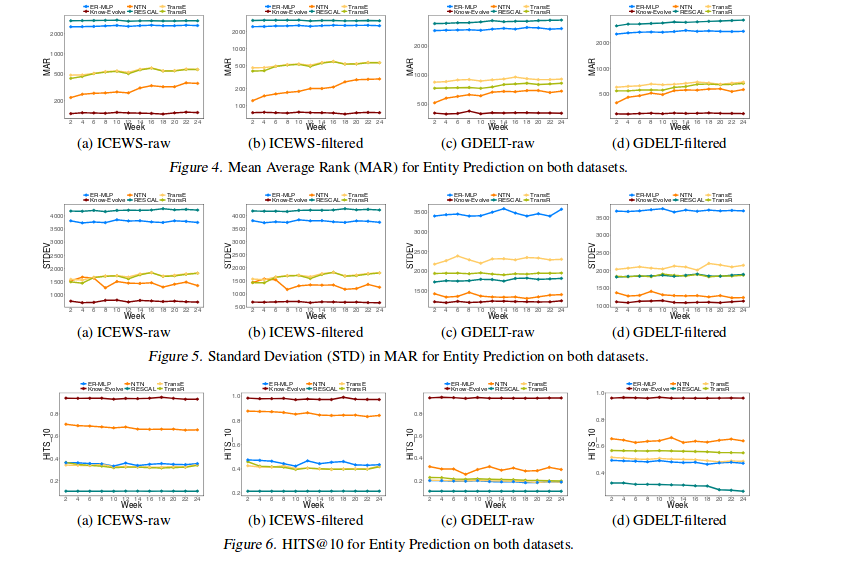## 前言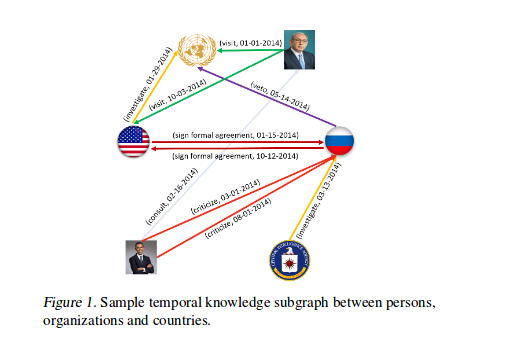### 时间点过程

$f(t) = \lambda (t) s(t)$

$s(t|T) = exp(-\int_{t_n}^{t} \lambda (\tau) d\tau)$

### 时序知识图谱表示

$\lambda_{\tau}^{e^s,e^o}(t|\vec{t}) = f(g_{\tau}^{e^s,e^o}(\vec{t})) * (t - \vec{t})$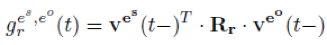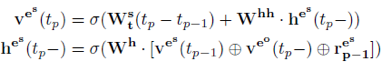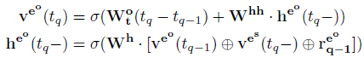### Know-Evolve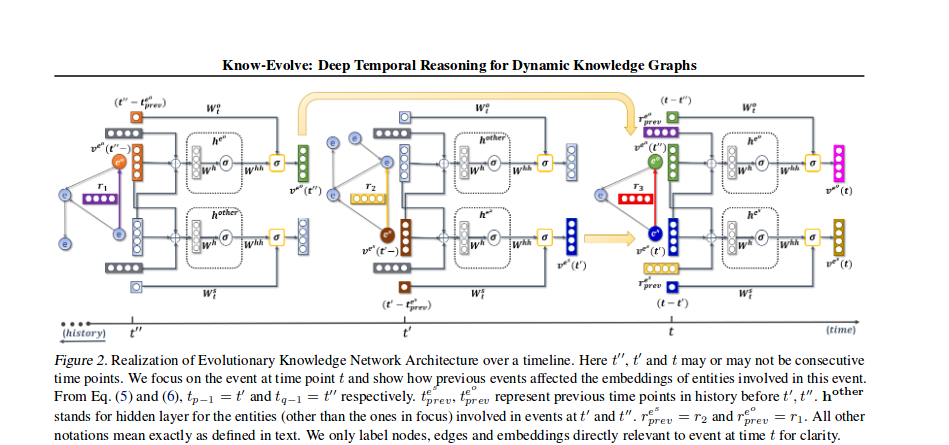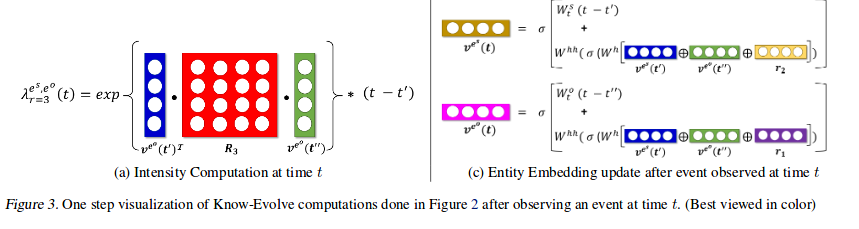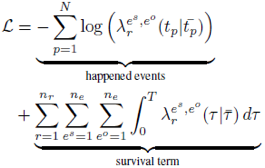### 实验